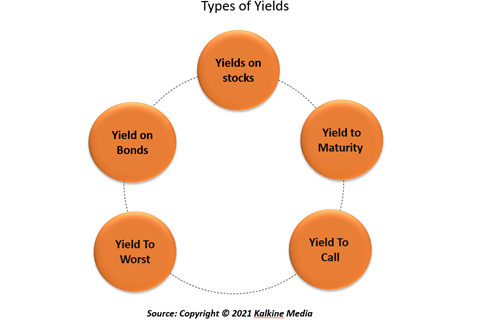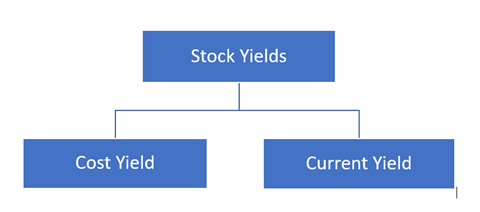# Yield

• Updated on

## What is Yield?

The term Yield refers to the income generated from an investment during a particular period. Yield is mainly reflected in percentage and is calculated by dividing dividends, coupons, or net income by the value of an investment.

It measures the income generated from an investment in the form of interest and dividends, ignoring the capital gains. Depending on whether the security owned by an investor is fixed or volatile, yields can either be classified as expected or anticipated.

Fluctuating securities are more susceptible to rate change or change in value. Therefore, it is usually seen that investments that are more exposed to fluctuations offer a higher rate of returns.

Summary
• Yield is a measure of dividends earned from an investment or interest earned from holding security without considering the capital gains.
• Yield is mainly reflected in percentage and is calculated by dividing dividends, coupons, or net income by the value of an investment.
• It should be remembered that there is a difference between Yield and total return as the latter is used to measure how much an investment earns or losses over time.

## How do we calculate Yield?

Yield is the income generated (excluding capital gains) in the form of interest or dividends from an investment in a security. It is mainly calculated on a yearly basis; however, as per the requirement, yields are also calculated quarterly and monthly. It should be remembered that there is a difference between Yield and total return as the latter is used to measure how much an investment earns or losses over time.

The formula to calculate Yield is:For an investor, the gains and return from investment on stocks can come in two ways. First, when an investor buys a stock at \$200 per share in the case of a price rise and after 12 months, they are sold at \$220. Second, let us assume that the stock pays a dividend of \$4 per share for that period. For this investment, the Yield would be the sum of the appreciation in the share price and the dividend paid, divided by the initial price of the stock.

(\$20 + \$4) / \$200 = 0.12, or 12%

## How can one obtain a higher Yield from an investment?Image Source: © Yeyendesign | Megapixl.com

A higher yield indicates that the investor would generate more earnings or cash flows from an investment. One will obtain a higher yield value if the market value of the security has fallen. Once the original value of the security, which is in the denominator, reduces, there is an increase in the yields from an investment. Investors, at times, lay focus on dividend payments they receive from stocks. However, it is equally important to observe the yields from an investment. This is because a very high yield would indicate two things; i) a decline in stock prices, and second ii) the company is paying high dividends.

A company pays dividends from its earnings. Higher dividend payouts indicate that a company's earnings are on the rise, which results in higher stock prices.

Furthermore, a considerable increase in Yield when the stock prices are unchanged would mean that a company is paying the dividend to the investors even when there is no increase in its earnings. In such a situation, a company is more likely to face cash flow problems soon.

## What are the different types of Yields?Yields can be of various types based on the type of security where investments are made, return from an investment and duration of investment.

1. Yield on Stocks

For stock-related investments, there are two types of yields.1. Cost Yield:When the calculation is based on the original price of the security, it is known as Yield on cost (YOC), or cost yield.

The formula to calculate cost yield is:Assume that an investor earns a profit of \$20 (\$220 - \$200) due to a price rise in stock. This is in addition to the \$4 paid by the company in the form of a dividend. The cost yield for this will be (\$20 + \$4) / \$200 = 0.12, or 12%1. Current Yield:Investors who, instead of the purchase price, use the current market price to calculate the Yield from an investment in stocks.

The formula to calculate the current Yield is:Thus, the current yield for the above example will be to (\$20 + \$4) / \$220 = 10.90%

It is important to note that there is an inverse relationship between the stock price and Yield. Therefore, an increase in a company's stock price would indicate a lower yield.

1. Yield on Bonds

The method used to calculate the Yield on bonds that pay annual interest to investors on investments made by them is referred to as nominal Yield.

The formula to calculate nominal Yield is:If an investor has invested money on a Treasury bond with a par value of \$2,000 with a maturity of 1 year and pays an annual interest of 5%. Then the yield from this treasury bond will be \$100 / \$2,000 = 0.05 or 5%.

For floating rate bonds, which provide different interest rates to the bondholder over their tenure, the Yield will change depending upon the different interest rates applicable at different times.

1. Yield to Maturity

Yield to maturity (YTM) is a measure of rate of return that an investor is likely to earn annually if they hold a bond until the security is matured. The value of YTM is mostly the same throughout the holding period until the bond has reached its full value or has matured.

1. Yield to Worst (YTW)

The remaining two types of yields are: Yield to Worst (YTW) and Yield to Call (YTC). Yield To Worst refers to the minimum possible Yield that an investor can receive from a bond, assuming that the bond issuer will not default on its payments. With the help of YTW, investors have a fair idea about how their future earnings will be impacted in the worst-case scenario.

1. Yield to Call (YTC)

Yield To Call refers to the amount paid to the bondholder if the issuer of a callable bond decides to pay it off before the bond's maturity.

##### Top ASX Listed Companies

Definition:
We use cookies to ensure that we give you the best experience on our website. If you continue to use this site we will assume that you are happy with it. OK